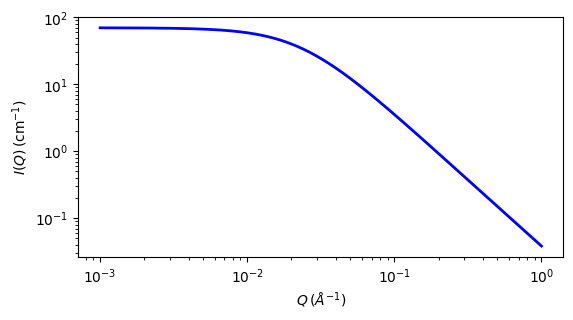# poly_gauss_coil

Scattering from polydisperse polymer coils

Parameter

Description

Units

Default value

scale

Scale factor or Volume fraction

None

1

background

Source background

cm-1

0.001

i_zero

Intensity at q=0

cm-1

70

rg

75

polydispersity

Polymer Mw/Mn

None

2

The returned value is scaled to units of cm-1 sr-1, absolute scale.

This empirical model describes the scattering from polydisperse polymer chains in theta solvents or polymer melts, assuming a Schulz-Zimm type molecular weight distribution.

To describe the scattering from monodisperse polymer chains, see the mono_gauss_coil model.

Definition

$I(q) = \text{scale} \cdot I_0 \cdot P(q) + \text{background}$

where

$\begin{split}I_0 &= \phi_\text{poly} \cdot V \cdot (\rho_\text{poly}-\rho_\text{solv})^2 \\ P(q) &= 2 [(1 + UZ)^{-1/U} + Z - 1] / [(1 + U) Z^2] \\ Z &= [(q R_g)^2] / (1 + 2U) \\ U &= (Mw / Mn) - 1 = \text{polydispersity ratio} - 1 \\ V &= M / (N_A \delta)\end{split}$

Here, $$\phi_\text{poly}$$, is the volume fraction of polymer, $$V$$ is the volume of a polymer coil, $$M$$ is the molecular weight of the polymer, $$N_A$$ is Avogadro’s Number, $$\delta$$ is the bulk density of the polymer, $$\rho_\text{poly}$$ is the sld of the polymer, $$\rho_\text{solv}$$ is the sld of the solvent, and $$R_g$$ is the radius of gyration of the polymer coil.

The 2D scattering intensity is calculated in the same way as the 1D, but where the $$q$$ vector is redefined as

$q = \sqrt{q_x^2 + q_y^2}$Fig. 114 1D plot corresponding to the default parameters of the model.

Source

poly_gauss_coil.py

References

1. O Glatter and O Kratky (editors), Small Angle X-ray Scattering, Academic Press, (1982) Page 404

2. J S Higgins, H C Benoit, Polymers and Neutron Scattering, Oxford Science Publications, (1996)

3. S M King, Small Angle Neutron Scattering in Modern Techniques for Polymer Characterisation, Wiley, (1999)

4. http://www.ncnr.nist.gov/staff/hammouda/distance_learning/chapter_28.pdf

Authorship and Verification

• Author: